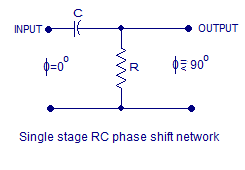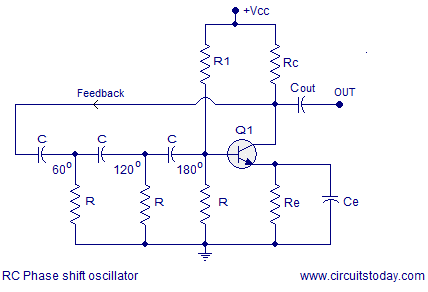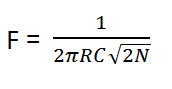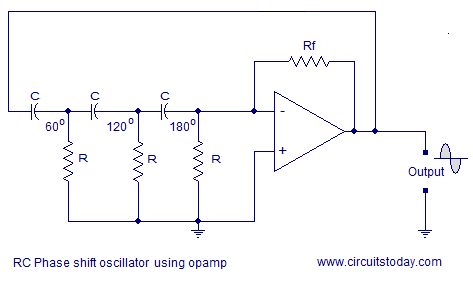## Audio

[Audio][slideshow]

### RC Phase shift oscillator.

RC phase shift oscillator or simply RC oscillator is a type of oscillator where a simple RC network (resistor-capacitor) network is used for giving the required phase shift to the feedback signal. In LC oscillators like Hartley oscillator and Colpitts oscillator an LC network (inductor- capacitor network) is used for providing the necessary positive feedback. The main feature of an RC phase shift oscillator is the excellent frequency stability. The RC oscillator can output a pure sine wave on a wide range of loads.

##### RC phase shift network.

RC phase shift network is a simple resistor capacitor network that can be used to give a desired phase shift to a signal. The circuit diagram of a simple single stage RC network is shown in the figure below.Theoretically in a simple RC circuit , the output voltage will lead the input voltage by a phase angle Φ =90°. Anyway in practical case the phase angle will be something below 90° just because it is impossible to get a purely ideal capacitor. Phase shift of a practical RC network depends on the value of the capacitor, resistor and the operating frequency.

Let F be the operating frequency, R be the resistance and C be the capacitance. Then the capacitive reactance Xc to the frequency F can be given by the equation Xc = 1 / (2πFC)

The effective impedance of the circuit can be given by the equation Z = √( R² + Xc²)

The phase angle of the RC network can be derived as Φ = tan-1 (Xc/R)

Just by making an RC network with phase shift equal to 60° and cascading three of them together the desired phase shift of 180° can be attained. This 180° phase shift by the RC network plus the 180° phase shift made by the transistor gives a total phase shift of 360° between the input and output which is the necessary condition for maintaining sustained oscillations. The circuit diagram of a three stage RC network producing a phase shift of 180° is shown in the figure below.Connecting such a three stage RC phase shift network between the input and output of a common emitter transistor amplifier will result in a transistor based RC phase shift oscillator. The circuit diagram is shown below.In the circuit diagram resistor R1 and the resistor R (close to the base of Q1 in the diagram) gives a voltage divider bias to the transistor Q1. Resistor Rc limits the collector current while Re is meant for thermal stability. Ce is the emitter by-pass capacitor and Cout is the output DC decoupling capacitor. By using more than three RC phase shift stages (like 4 x 45°) the frequency stability of the oscillator can be further improved. The frequency of the transistor RC phase shift oscillator oscillator can be expressed by the equation:Where F is the frequency, R is the resistance, C is the capacitance and N is the number of RC phase shift stages. The RC pahse shift oscillator can be made variable by making the resistors or capacitors variable. The common approach is to leave the resistors untouched the three capacitors are replaced by a triple gang variable capacitor.

#### RC phase shift oscillator using opamp.The circuit diagram of an RC phase shift oscillator is shown above. Feedback resistor Rf and resistor R (close to the inverting pin of the opamp in the circuit) is used to set the gain of the oscillator. Three stage phase shift network comprising of R and C produces 180° phase shift and the opamp itself wired in inverting mode produces another 180° phase shift. Thus the required phase shift of 360° is obtained between input and output.

[Lights][grids]